Home MathLab Matlab Matrix Operations – Exercise

# Matlab Matrix Operations – Exercise

Lately we have learned some basics about Matlab matrix operations. Here come the part 2 of that post, where we will test our abilities of manipulating matrices in Matlab

## Matlab matrix operation exercise

### Exercise 1

Create a 1 x 5 vector A with all elements equal to 0

### Exercise 2

Create a 3 x 1 vector B with with all elements equal to 1

### Exercise 3

Create a 1 x 5 vector C with elements equal to 1, 2, 3, 4, π respectively.

### Exercise 4

Create 1-row vector D with element’s from 3 to 27 with step 3 by using the appropriate operator

### Exercise 5

Create a 3 x 3 matrix E with elements with random values.

### Exercise 6

Create the following matrix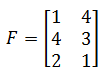### Exercise 7

Check the size of F

### Exercise 8

Create the following Matrix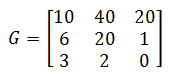1. find the transpose of G
2. print on display the element G13 of the transpose of G
3. print on display the second row of the transpose of G
4. print on display the third column of the transpose of G
5. find the determinant of G

### Exercise 9

Create the following Matrices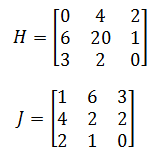1. Find the Matrix H+J
2. Find the Matrix H * J
3. Multiply H by J, element-by-element

### Exercise 10

Solve the linear system of equations ax=b if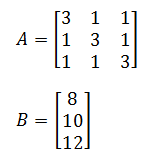### Exercise 11

1. Create a 1 x 5 vector with elements with random values
2. Use the “for” loop to find the greatest element of the matrix
3. Use the “while” loop to find the smallest element of the matrix

### Exercise 12

Use the diag operator to generate the matrix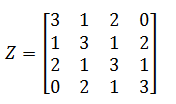### Exercise 13

Let’s consider the following vector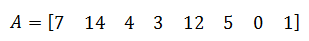1. Define a vector K, K being the vector A sorted in ascending order
2. Define a vector L, L being the vector A sorted in Descending order